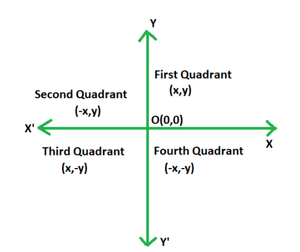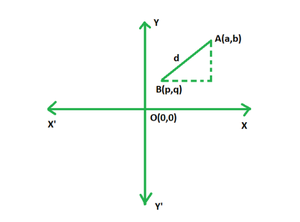GeeksforGeeks App
Open AppBrowser
Continue

# Find a relation between x and y if the point (x, y) is equidistant from (3, 6) and (-3, 4)

Geometry is one of the oldest fields of mathematics, with a wide range of applications and influences on our daily lives. Geometry is a discipline of mathematics that studies the proportions, sizes, dimensions, forms, shapes, and angles of many objects in our environment. All the objects around us have a distinct shape, occupy a substantial amount of space, can be used to store a specific amount of items, and maybe positioned in various ways. Geometry encompasses all of these variables. Two-dimensional and three-dimensional shapes are the two types of shapes.

### Cartesian Plane

The coordinate plane and cartesian system are two of the most important topics in geometry. When one horizontal and one vertical line connect and form four quadrants, a cartesian plane or coordinate plane is formed. Different points can be represented by each quadrant. Each quadrant’s coordinates can be used to represent distinct points.Distance Formula

The distance formula is used when calculating how far apart two given points are on a cartesian plane. The diagram below shows point A(a, b) and B(p, q). For calculating the distance between them, we need to find the length AB. Pythagoras Theorem can be conveniently applied in order to calculate the required length. In fact, distance formula is a direct application of the Pythagorean Theorem.The distance between any two points A(a, b) and B(p, q) on the cartesian plane is given by:

D =Thus the distance between two points on a cartesian plane is equal to the square root of the total sum of the squares of the difference between their respective x and y coordinates.

### Find a relation between x and y if the point (x, y) is equidistant from (3, 6) and (-3, 4)

Solution:

Given: Point P(x, y) is equidistant from both A(3, 6) and B(-3, 4).

Using distance formula, distance between P(x, y) and A(3, 6) is given by:

D1Using distance formula, distance between P(x, y) and B(-3, 4) is given by:

D2Since it is given that D1 = D2, we have:Square both sides. Then,

x2 + 9 – 6x + y2 + 36 – 12y = x2 + 9 + 6x + y2 + 16 – 8y

⇒ 12x + 4y = 20

⇒ 3x + y = 5

⇒ 3x + y – 5 = 0

### Similar Problems

Question 1. Find x if the point (0, 1) is equidistant from (x, 6) and (5, -3)?

Solution:

Given: Point P(0, 1) is equidistant from both A(x, 6) and B(5, -3).

Using distance formula, distance between P(0, 1) and A(x, 6) is given by:

D1 =Using distance formula, distance between P(0, 1) and B(5, -3) is given by:

D2 =Since it is given that D1 = D2, we have:Square both sides. Then,

x = −4

Question 2. What is the relation between X and Y if the point (x, y) is equidistant from (1, 2) and (3, 5)?

Solution:

Given: Point P(x, y) is equidistant from both A(1, 2) and B(3, 5).

Using distance formula, distance between P(x, y) and A(1, 2) is given by:

D1Using distance formula, distance between P(x, y) and B(3, 5) is given by:

D2Since it is given that D1 = D2, we have:Square both sides. Then,

x2 + 1 – 2x + y2 + 4 – 4y = x2 + 9 – 6x + y2 + 25 – 10y

⇒ 6x – 2x + 10y – 4y = 9 – 1 + 25 – 4

⇒ 4x + 6y = 29

Question 3. Find y if the distance between the points P(2, – 3) and Q(10, y) is 10 units.

Solution:

Given: PQ = 10 units

Using distance formula, we have:⇒Square both sides.

⇒ 64 + (y +3)2 = 100

⇒ (y +3)2 = 36

⇒ y = 3 or −9

Question 4. What is the relation between X and Y if the point (x, y) is equidistant from (3, 6) and (-3, 5)?

Solution:

Given: Point P(x, y) is equidistant from both A(3, 6) and B(-3, 5).

Using distance formula, distance between P(x, y) and A(3, 6) is given by:

D1Using distance formula, distance between P(x, y) and B(-3, 5) is given by:

D2Since it is given that D1 = D2, we have:Square both sides. Then,

x2 + 9 – 6x + y2 + 36 – 12y = x2 + 9 + 6x + y2 + 25 – 10y

⇒ 6x + 6x + 12y – 10y = 36 – 25

⇒ 12x + 2y = 11

Question 5. What is the relation between X and Y if the point (x, y) is equidistant from (3, 6) and (-3, 2)?

Solution:

Given: Point P(x, y) is equidistant from both A(3, 6) and B(-3, 2).

Using distance formula, distance between P(x, y) and A(3, 6) is given by:

D1Using distance formula, distance between P(x, y) and B(-3, 2) is given by:

D2Since it is given that D1 = D2, we have:Square both sides. Then,

x2 + 9 – 6x + y2 + 36 – 12y = x2 + 9 + 6x + y2 + 4 – 4y

⇒ 12x – 8y = -32

⇒ 3x – 2y = -8

My Personal Notes arrow_drop_up
Related Tutorials